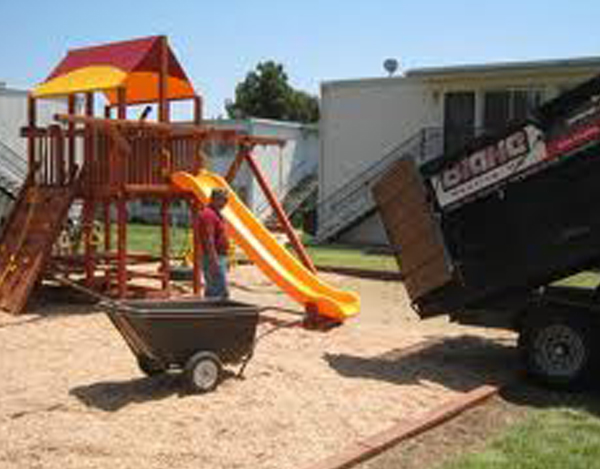Select PageDivide the beds into squares, rectangles, triangles & circles to make it easier to calculate.

• To find the area of a SQUARE – multiply the length x’s the width
• To find the area of a RECTANGLE – multiply the length x’s the width
• To find the area of a CIRCLE – multiply 3.14 x radius x radius (or r2)
• To find the area of a TRIANGLE – multiply 1/2 x the base x the height
• Add the totals together to get your total square footage = “M”
• Convert to square footage to cubic yards
• Take your total square footage (M) and divide by 120 to get “C”

Example 1,200 sq ft / 120 = 10 cubic yards